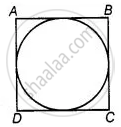# The area of the circle that can be inscribed in a square of side 6 cm, is ______. - Mathematics

MCQ
Fill in the Blanks

The area of the circle that can be inscribed in a square of side 6 cm, is ______.

• 36π cm2

• 18π cm2

• 12π cm2

• 9π cm2

#### Solution

The area of the circle that can be inscribed in a square of side 6 cm, is underline(bb(9 pi  "cm"^2)).

Explanation:Given, side of square = 6 cm

∴ Diameter of a circle, (d) = Side of square = 6 cm

∴ Radius of a circle (r) = d/2 = 6/2 = 3 cm

∴ Area of circle = π(r)2

= π(3)2

= 9π cm2

Concept: Area of Circle
Is there an error in this question or solution?

#### APPEARS IN

NCERT Mathematics Exemplar Class 10
Chapter 11 Area Related To Circles
Exercise 11.1 | Q 7 | Page 121

Share